# Java Program to add two binary numbers

In this tutorial you will learn about the Java Program to add two binary numbers and its application with practical example.

In this tutorial, we will learn to create a Java Program to Add two Binary Numbers in Java using Java programming.

## Prerequisites

Before starting with this tutorial we assume that you are best aware of the following Java programming topics:

• Java Operators.
• Basic Input and Output function in Java.
• Class and Object in Java.
• Basic Java programming.
• If-else statements in Java.
• For loop in Java.
• inbuilt Function

## Binary Number System.

A Number system in which  numbers are represented by using only two digits (0 and 1) with a base 2 is called a binary number system.

For Binary addition you must remember the following  rules
The four rules of binary addition are:

• 0 + 0 = 0
• 0 + 1 = 1
• 1 + 0 = 1
• 1 + 1 =10.

Just look at the table how we add values in Binary are shown.

## x+y

0                     0                                                           0
0                     1                                                          1
1                     0                                                          1
1                     1                                                          0      (where 1 is carried over)

## Java Program to add two binary numbers

In this program we will learn to add two binary numbers.First of all we declare required variable and also initiates required values in variable. Next we take value from user at run time and then after we will add two binary given by user.

## Output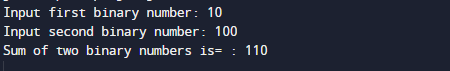In the above program, we have first declared and initialized a set variables required in the program.

• no1 = it will hold first given binary number.
• no2= it will hold second Binary number.
• sum= it will hold sum of numbers.
• rem= ot will hold reminder of values.

First of all we declared and initialized set of variables required in the program. Then we will take a value from user at run time as shown in image below.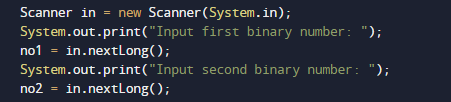After taking all the values for user we will perform Binary Addition using the following formula.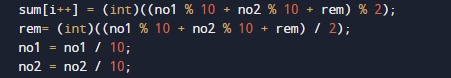With in the while loop first of all we check the given number are greater than zero and after that with all the four rule we add two given number by user as shown below.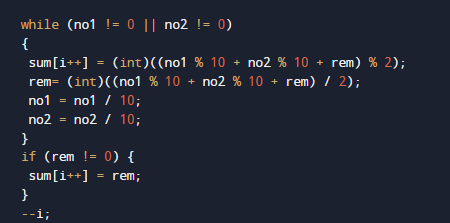User enter the two binary numbers that we add bit by bit using while loop and storing the result in sum.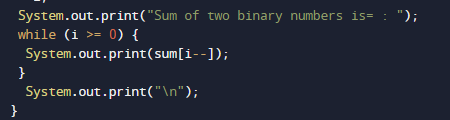And finally we will print the result.

In this tutorial we have learn about the Java Program to add two binary numbers and its application with practical example. I hope you will like this tutorial.